# TEXTJOIN function in Excel to merge text from multiple cells into one

The tutorial shows how to use the TEXTJOIN function to merge text in Excel with practical examples.

Until recently, there were two prevalent methods to merge cell contents in Excel: the concatenation operator and CONCATENATE function. With the introduction of TEXTJOIN, it seems like a more powerful alternative has appeared, which enables you to join text in a more flexible manner including any delimiter in between. But in truth, there's much more to it!

## Excel TEXTJOIN function

TEXTJOIN in Excel merges text strings from multiple cells or ranges and separates the combined values with any delimiter that you specify. It can either ignore or include empty cells in the result.

The function is available in Excel for Office 365, Excel 2021, and Excel 2019.

The syntax of the TEXTJOIN function is as follows:

TEXTJOIN(delimiter, ignore_empty, text1, [text2], …)

Where:

• Delimiter (required) - is a separator between each text value that you combine. Usually, it is supplied as a text string enclosed in double quotes or a reference to a cell containing a text string. A number supplied as a delimiter is treated as text.
• Ignore_empty (required) - Determines whether to ignore empty cells or not:
• TRUE - ignore any blank cells.
• FALSE - include empty cells in the resulting string.
• Text1 (required) - first value to join. Can be supplied as a text string, a reference to a cell containing a string, or array of strings such as a range of cells.
• Text2, … (optional) - additional text values to be joined together. A maximum of 252 text arguments are allowed, including text1.

As an example, let's combine address parts from cells B2, C2 and D2 together into one cell, separating the values with a comma and a space:

With the CONCATENATE function, you'd need to specify each cell individually and put a delimiter (", ") after each reference, which might be bothersome when merging the contents of many cells:

`=CONCATENATE(A2, ", ", B2, ", ", C2)`

With Excel TEXTJOIN, you specify the delimiter just once in the first argument, and supply a range of cells for the third argument:

`=TEXTJOIN(", ", TRUE, A2:C2)`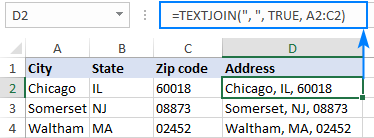### TEXTJOIN in Excel - 6 things to remember

To effectively use TEXTJOIN in your worksheets, there are a few important points to take notice of:

1. TEXTJOIN is a new function, which is only available in Excel 2019 - Excel 365. In earlier Excel versions, please use the CONCATENATE function or the "&" operator instead.
2. In new versions if Excel, you can also use the CONCAT function to concatenate values from separate cells and ranges, but with no options for delimiters or empty cells.
3. Any number supplied to TEXTJOIN for the delimiter or text arguments is converted to text.
4. If delimiter is not specified or is an empty string (""), text values are concatenated without any delimiter.
5. The function can handle up to 252 text arguments.
6. The resulting string can contain a maximum of 32,767 characters, which is the cell limit in Excel. If this limit is exceeded, a TEXTJOIN formula returns the #VALUE! error.

## How to join text in Excel - formula examples

To better understand all the advantages of TEXTJOIN, let's take a look at how to use the function in real-life scenarios.

### Convert column to comma separated list

When you are looking to concatenate a vertical list separating the values by a comma, semicolon or any other delimiter, TEXTJOIN is the right function to use.

For this example, we'll be concatenating wins and losses of each team from the table below. This can be done with the following formulas, which differ only in the range of cells that are joined.

For Team 1:

`=TEXTJOIN(",", FALSE, B2:B6)`

For Team 2:

`=TEXTJOIN(",", FALSE, C2:C6)`

And so on.

In all the formulas, the following arguments are used:

• Delimiter - a comma (",").
• Ignore_empty is set to FALSE to include empty cells because we need to show which games were not played.

As the result, you will get four comma-separated lists that represent wins and losses of each team in a compact form: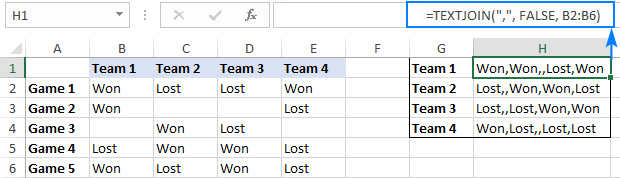### Join cells with different delimiters

In a situation when you need to separate the combined values with different delimiters, you can either supply several delimiters as an array constant or input each delimiter in a separate cell and use a range reference for the delimiter argument.

Supposing you want to join cells containing different name parts and get the result in this format: Last name, First name Middle name.

As you can see, the Last name and First name are separated by a comma and a space (", ") while the First name and Middle name by a space (" ") only. So, we include these two delimiters in an array constant {", "," "} and get the following formula:

`=TEXTJOIN({", "," "}, TRUE, A2:C2)`

Where A2:C2 are the name parts to be combined.

Alternatively, you can type the delimiters without quotation marks in some empty cells (say, a comma and a space in F3 and a space in G3) and use the range \$F\$3:\$G\$3 (please mind the absolute cell references) for the delimiter argument:

`=TEXTJOIN(\$F\$3:\$G\$3, TRUE, A2:C2)`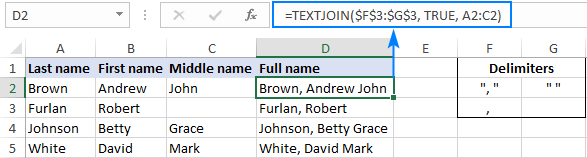By using this general approach, you can merge cell contents in various forms.

For example, if you want the result in the First name Middle initial Last name format, then use the LEFT function to extract the first character (the initial) from cell C2. As for the delimiters, we put a space (" ") between the First name and the Middle initial; a period and a space (". ") between the Initial and the Last name:

`=TEXTJOIN({" ",". "}, TRUE, B2, LEFT(C2,1), A2)`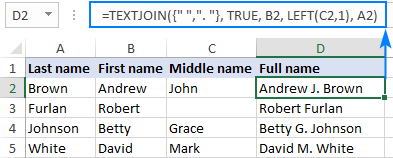### Join text and dates in Excel

In a specific case when you are merging text and dates, supplying dates directly to a TEXTJOIN formula won't work. As you may remember, Excel stores dates as serial numbers, so your formula will return a number representing the date as shown in the screenshot below:

`=TEXTJOIN(" ", TRUE, A2:B2)`To fix this, you need to convert the date into a text string before joining it. And here the TEXT function with the desired format code ("mm/dd/yyyy" in our case) comes in handy:

`=TEXTJOIN(" ", TRUE, A2, TEXT(B2, "mm/dd/yyyy"))`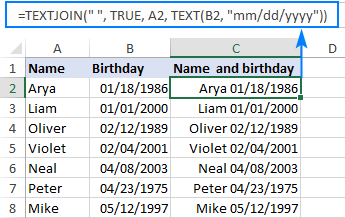### Merge text with line breaks

If you'd like to merge text in Excel so that each value starts in a new line, use CHAR(10) as the delimiter (where 10 is a linefeed character).

For example, to combine text from cells A2 and B2 separating the values by a line break, this is the formula to use:

`=TEXTJOIN(CHAR(10), TRUE, A2:B2)`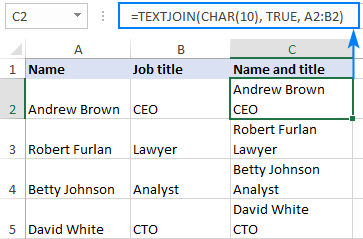Tip. For the result to display in multiple lines like shown in the screenshot above, make sure the Wrap text feature is turned on.

### TEXTJOIN IF to merge text with conditions

Due to the ability of Excel TEXTJOIN to handle arrays of strings, it can also be used to conditionally merge the contents of two or more cells. To have it done, use the IF function to evaluate a range of cells and return an array of values that meet the condition to the text1 argument of TEXTJOIN.

From the table shown in the screenshot below, suppose you wish to retrieve a list of Team 1 members. To achieve this, nest the following IF statement into the text1 argument:

`IF(\$B\$2:\$B\$9=1, \$A\$2:\$A\$9, "")`

In plain English, the above formula says: If column B equals 1, return a value from column A in the same row; otherwise return an empty string.

The complete formula for Team 1 takes this shape:

`=TEXTJOIN(", ", TRUE, IF(\$B\$2:\$B\$9=1, \$A\$2:\$A\$9, ""))`

In a similar manner, you can get a comma-separated list of the members of Team 2:

`=TEXTJOIN(", ", TRUE, IF(\$B\$2:\$B\$9=2, \$A\$2:\$A\$9, ""))`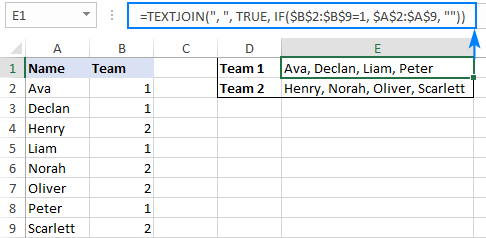Note. Due to the Dynamic Arrays feature available in Excel 365 and 2021, this works as a regular formula, shown in the screenshot above. In Excel 2019, you must enter it as a traditional array formula by pressing the Ctrl + Shift + Enter shortcut.

### Lookup and return multiple matches in comma separated list

As you probably know, the Excel VLOOKUP function can only return the first found match. But what if you need to get all matches for a specific ID, SKU, or something else?

To output the results in separate cells, use one of the formulas described in How to VLOOKUP multiple values in Excel.

To look up and return all matching values in a single cell as a comma-separated list, use the TEXTJOIN IF formula.

To see how it works in practice, let's retrieve a list of products purchased by a given seller from the sample table below. This can be easily done with the following formula:

`=TEXTJOIN(", ", TRUE, IF(\$A\$2:\$A\$12=D2, \$B\$2:\$B\$12, ""))`

Where A2:A12 are seller names, B2:B12 are products, and D2 is the seller of interest.

The above formula goes to E2 and brings all the matches for the target seller in D2 (Adam). Due to the clever use of relative (for the target seller) and absolute (for the seller names and products) cell references, the formula correctly copies to the below cells and works nicely for the other two sellers too: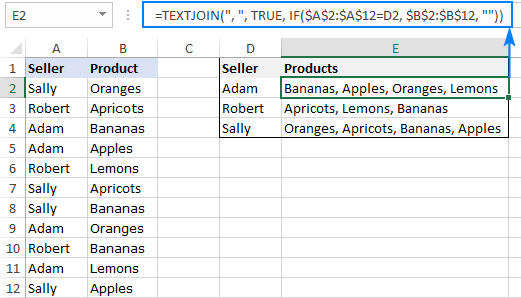Note. As with the previous example, this works as a regular formula in Excel 365 and 2021, and as a CSE formula (Ctrl + Shift + Enter ) in Excel 2019.

The formula's logic is exactly the same as in the previous example:

The IF statement compares each name in A2:A12 against the target name in D2 (Adam in our case):

`IF(\$A\$2:\$A\$12=D2, \$B\$2:\$B\$12, "")`

If the logical test evaluates to TRUE (i.e. the name in D2 matches the name in column A), the formula returns a product from column B; otherwise an empty string ("") is returned. The result of IF is the following array:

`{"";"";"Bananas";"Apples";"";"";"";"Oranges";"";"Lemons";""}`

The array goes to the TEXTJOIN function as the text1 argument. And because TEXTJOIN is configured to separate the values with a comma and a space (", "), we get this string as the final result:

Bananas, Apples, Oranges, Lemons

## Excel TEXTJOIN not working

When your TEXTJOIN formula results in an error, it's most likely to be one of the following:

• #NAME? error occurs when TEXTJOIN is used in an older version of Excel where this function is not supported (pre-2019) or when the function's name is misspelled.
• #VALUE! error occurs if the resulting string exceeds 32,767 characters.
• #VALUE! error may also occur if Excel does not recognize the delimiter as text, for example if you supply some non-printable character such as CHAR(0).

That's how to use the TEXTJOIN function in Excel. I thank you for reading and hope to see you on our blog next week!